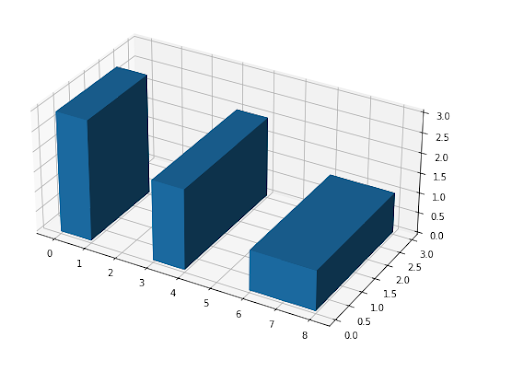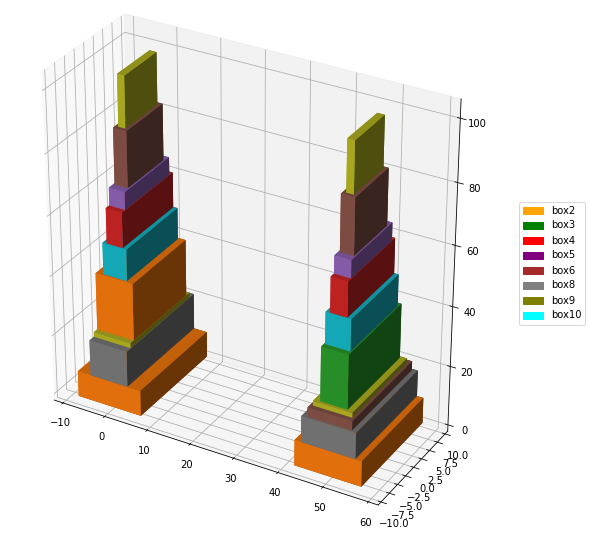## Saturday, October 16, 2021

### Stacking 3d boxes under rotationBoston custom house tower

Assume we have an infinite collection of 3d boxes of different sizes. As an example let's use :

----     30 PARAMETER boxes  available box sizes

size-x      size-y      size-z

box1           4           6           7
box2           1           2           3
box3           4           5           6
box4          10          12          32


We want to build a tower of boxes such that the base of the box on top is strictly smaller than the underlying box. The "strictly" part is essential. Otherwise, we can just repeat the same type of box ad infinitum. The interesting angle is: we can rotate the boxes. Without loss of generality, we can assume that for all boxes, after rotation, we have: the width ($$x$$) is less than the depth $$(y$$). (This may require a bit of thinking). With this, any box can be placed in just three different ways.3d rotations

The objective is to build a tower that is as tall as possible. In , this problem is solved using a Dynamic Programming algorithm. Here I want to see how this looks using a MIP model.

#### Model development

The first thing to do is to create the rotations. I created a mapping set that maps from one size to another. Then I applied this mapping to all our boxes. The result is:

----     38 SET rot  rotate s1->s2

size-x      size-y      size-z

rot1.size-x         YES
rot1.size-y                     YES
rot1.size-z                                 YES
rot2.size-x         YES
rot2.size-y                                 YES
rot2.size-z                     YES
rot3.size-x                                 YES
rot3.size-y         YES
rot3.size-z                     YES

----     44 PARAMETER sizes  sizes under rotation

size-x      size-y      size-z

box1.rot1           4           6           7
box1.rot2           4           7           6
box1.rot3           6           7           4
box2.rot1           1           2           3
box2.rot2           1           3           2
box2.rot3           2           3           1
box3.rot1           4           5           6
box3.rot2           4           6           5
box3.rot3           5           6           4
box4.rot1          10          12          32
box4.rot2          10          32          12
box4.rot3          12          32          10


I used the assumption that the original data is ordered by size (i.e. $$x \le y \le z$$). See our first data table above to verify that this indeed holds. The set $$\color{darkblue}{\mathit{rot}}$$ was created by hand: it says what type of rotations we can apply. It produces three rotations as follows:$\begin{pmatrix}x\\y\\z\end{pmatrix} \rightarrow \begin{pmatrix}x\\y\\z\end{pmatrix}\> \begin{pmatrix}x\\z\\y\end{pmatrix}\>\begin{pmatrix}y\\z\\x\end{pmatrix}$ Note that all these rotations obey that the first coordinate is less-than-or-equal to the second coordinate. After this, we just apply this operation to our original data table and produce all rotations for all boxes in one swoop.

With this under our belt, the model is not very difficult. We use the following indices:

• $$i$$: the level. Just use a set that is larger than the number of levels you expect.
• $$k$$: the box type from the inventory.
• $$r$$: the rotation
• $$s$$: the sizes in each direction.
• $${\mathit{xy}}$$: subset of $$s$$, only the $$x$$ and $$y$$ coordinates.

I use the following variables:\begin{aligned}\color{darkred}{\mathit{assign}}_{i,k,r} &= \begin{cases} 1 & \text{if box k under rotation r is assigned to level i} \\ 0 & \text{otherwise}\end{cases} \\ \color{darkred}b_i &= \begin{cases} 1 & \text{if a box is assigned to level i}\\ 0 & \text{otherwise}\end{cases}\\ \color{darkred}{\mathit{size}}_{i,s} &= \text{the size of the box at level i (or zero)} \end{aligned} The rotation index $$r$$ essentially gives us three times as many boxes to choose from.

MIP Model
\begin{align}\max\>&\color{darkred}{\mathit{height}}=\sum_i \color{darkred}{\mathit{size}}_{i,z}\\ & \color{darkred}b_i = \sum_{k,r}\color{darkred}{\mathit{assign}}_{i,k,r} \\ & \color{darkred}b_i \le \color{darkred}b_{i-1} && \text{ordering} \\ & \color{darkred}{\mathit{size}}_{i,s} = \sum_{k,r}\color{darkred}{\mathit{assign}}_{i,k,r} \cdot \color{darkblue}{\mathit{sizes}}_{k,r,s} && \text{size at level i} \\ & \color{darkred}{\mathit{size}}_{i,xy} \le\color{darkred}{\mathit{size}}_{i-1,xy}-0.5\cdot\color{darkred}b_i && \text{smaller boxes on top} \end{align}

Notes:
• We start at the bottom, i.e. $$\color{darkred}b_1 = 1$$. So $$\color{darkred}b_i=0$$ only happens only at the top. The constraint $$\color{darkred}b_i \le \color{darkred}b_{i-1}$$ is not really needed as the equation that requires smaller boxes to be on top, has the same effect. So, we can make the model even more compact.
• We require that the width and the depth are both 0.5 less than the box of the previous layer. We make an exception when there is no box, in which case all sizes remain zero. If we have continuous sizes, we need to change this minimum shrinkage to something like 0.001 (this number should be larger than the feasibility tolerance of the solver).

This is a very small model. But it actually works. The solution looks like:

----     82 PARAMETER results  optimal stack

size-x      size-y      size-z

level1.box4.rot3          12          32          10
level2.box4.rot1          10          12          32
level3.box1.rot3           6           7           4
level4.box3.rot3           5           6           4
level5.box3.rot1           4           5           6
level6.box2.rot3           2           3           1
level7.box2.rot1           1           2           3


The total height is 60 (the sum of the $$z$$ column).

Notes:
• This model is of course very different from the Dynamic Programming algorithm shown in .
• It is very easy to change the model to use a maximum height in terms of blocks (instead of inches or cm). We can choose from:  \begin{align} & \max \> \color{darkred}{\mathit{height}}=\sum_i \color{darkred}{\mathit{size}}_{i,z} && \text{maximize height}\\ & \max\>\color{darkred}{\mathit{numBoxes}}=\sum_i \color{darkred}b_i && \text{maximize boxes (or levels)}\end{align} For this example, the solution is the same for both objectives.
• I started by saying we have an infinite supply of boxes. Actually, we never need more than two boxes of each type.

#### A larger data set

A slightly larger data set is:

----     34 PARAMETER boxes  available box sizes

size-x      size-y      size-z

box1        2.546      12.529      16.766
box2        8.589      14.798      18.961
box3        5.953      15.874      18.057
box4        3.710      11.570      13.921
box5        3.630       5.806      12.108
box6        3.016       9.774      18.252
box7        4.148       6.584       9.661
box8        8.706      11.957      18.949
box9        1.604       8.624      16.607
box10       5.502      10.420      14.153


This is using continuous sizes, and a minimum shrinkage threshold of $$\color{darkblue}\delta=0.001$$. Here we see a difference in the "maximum height" vs. the "maximum number of levels" objective. The results look like:

----    113 PARAMETER results  optimal stack

INDEX 1 = max height

size-x      size-y      size-z

level1.box2 .rot3      14.798      18.961       8.589
level2.box8 .rot2       8.706      18.949      11.957
level3.box9 .rot3       8.624      16.607       1.604
level4.box2 .rot1       8.589      14.798      18.961
level5.box10.rot2       5.502      14.153      10.420
level6.box4 .rot2       3.710      13.921      11.570
level7.box5 .rot2       3.630      12.108       5.806
level8.box6 .rot1       3.016       9.774      18.252
level9.box9 .rot1       1.604       8.624      16.607

INDEX 1 = max boxes

size-x      size-y      size-z

level1 .box2 .rot3      14.798      18.961       8.589
level2 .box8 .rot3      11.957      18.949       8.706
level3 .box6 .rot3       9.774      18.252       3.016
level4 .box9 .rot3       8.624      16.607       1.604
level5 .box3 .rot1       5.953      15.874      18.057
level6 .box10.rot2       5.502      14.153      10.420
level7 .box4 .rot2       3.710      13.921      11.570
level8 .box5 .rot2       3.630      12.108       5.806
level9 .box6 .rot1       3.016       9.774      18.252
level10.box9 .rot1       1.604       8.624      16.607

----    113 PARAMETER objectives  optimal values height vs boxes

height       boxes

max height     103.767       9.000
max boxes      102.629      10.000


In the picture below we see on the left the tower produced by maximizing the total height, while on the right the number of levels is maximized."max height" vs "max levels" results

This larger example also gives us the opportunity to see if the extra ordering condition $$\color{darkred}b_i \le \color{darkred}b_{i-1}$$ is helping or not. The model with the "max boxes" objective solves in zero nodes, so I'll focus on the "max height" objective.  I ran the "max height" problem both with and without the extra constraint, and the results are:

----    136 PARAMETER stats  statistics

iterations       nodes   objective

extra       2672.000     402.000     103.767
no extra    4679.000     713.000     103.767


I included the objective here just for checking. We see that including the ordering constraint can help performance.

#### Appendix: GAMS model

 $ontext stack boxes under rotation 1. Boxes on top should have a strictly smaller x/y base than the one underneath 2. Assume always: size x < size y 3. In size comparison I assume integer sizes, so we want to be at least 0.5 smaller.$offtext *-------------------------------------------------------------- * data *-------------------------------------------------------------- sets    s 'size' /size-x,size-y,size-z/    xy(s) /size-x,size-y/    r 'rotation' /rot1*rot3/    k 'box' /box1*box4/    i 'stack level' /level1*level10/ ; table boxes(k,s) 'available box sizes'         size-x   size-y   size-z box1       4        6        7 box2       1        2        3 box3       4        5        6 box4      10       12       32 ; option boxes:0; display boxes; scalar delta 'shrinkage in x,y directions' /0.5/; alias(s,s1,s2); *-------------------------------------------------------------- * derived data *-------------------------------------------------------------- set rot(r,s1,s2) 'rotate s1->s2' /    rot1.(size-x.size-x, size-y.size-y, size-z.size-z)    rot2.(size-x.size-x, size-y.size-z, size-z.size-y)    rot3.(size-x.size-z, size-y.size-x, size-z.size-y) /; display rot; parameter sizes(k,r,s) 'sizes under rotation'; sizes(k,r,s2) = sum(rot(r,s1,s2),boxes(k,s1)); option sizes:0; display sizes; *-------------------------------------------------------------- * model *-------------------------------------------------------------- binary variable    assign(i,k,r)  'assign (k,r) to level i'    b(i)           'level i has a box' ; variables    size(i,s) 'size of box at level i'    numboxes  'number of stacked boxes'    height    'total height of stack' ; equations    level(i)      'box per level'    order(i)      'below a box should be another box (optional: covered by eq. smaller)'    esize(i,s)    'size of box at level i'    smaller(i,xy) 'smaller boxes on top of large ones (xy only)'    countboxes    'number of boxes we can stack'    totalheight   'total height of the tower' ; level(i)..   b(i) =e= sum((k,r),assign(i,k,r)); order(i-1).. b(i) =l= b(i-1); esize(i,s).. size(i,s) =e= sum((k,r),assign(i,k,r)*sizes(k,r,s)); smaller(i-1,xy).. size(i,xy) =l= size(i-1,xy)-delta*b(i); countboxes.. numboxes =e= sum(i,b(i)); totalheight.. height =e= sum(i,size(i,'size-z')); model stackboxes /all/; option optcr=0; *-------------------------------------------------------------- * solve *-------------------------------------------------------------- *solve stackboxes maximizing numboxes using mip; solve stackboxes maximizing height using mip; *-------------------------------------------------------------- * reporting *-------------------------------------------------------------- parameter results(i,k,r,*) 'optimal stack'; results(i,k,r,s)\$(assign.l(i,k,r)>0.5) = size.l(i,s); option results:0:3:1; display results;

Notes:
• There are some interesting modeling issues in the model.
• The set levels should be larger than the expected size. (Obvious questions are: What happens if too small? Can we detect this?)
• The equation order is optional. It can help with large instances.
• Note how the equations order and smaller have protection against the index becoming 1. E.g. order(i-1). Check the equation listing to see the impact. (Question: what happens if we drop this?)